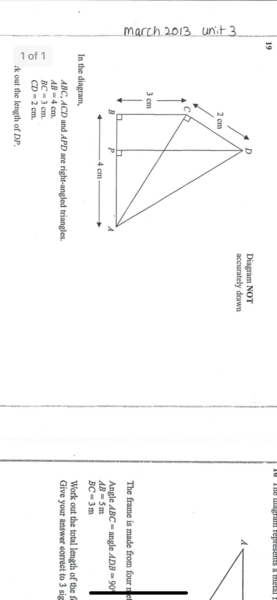or
to access all these features
or
to access all these features

Join the discussion and meet other Mumsnetters on our free online chat forum.

# Year 10 self isolation maths homework hell

4 replies

Choufleur · 26/10/2020 11:58

Can anybody help with ds’s trigonometry homework? I can’t remember how to do this 30 years after doing my GCSEs. TIAOP posts:
KihoBebiluPute · 27/10/2020 05:56

So firstly it is easy to work out AC using "the sum of the squares of the other two sides" and you know AB and BC.

Then you need to use trig.
Sin(x) = o/h
Cos(x) = a/h
Tan(x) = o/a (o = length of side opposite angle x, a = the other non-hypotenuse side and h is the hypotenuse)

So you can easily calculate angle BAC and CAD. Then add those two numbers to get angle BAD

Use pythagoras again to find the length of AD and you now know the value of an angle and the length of one side of triangle APD so you can use the Sin(x) law to calculate DP

Don't do it for him though! He needs to do the thinking himself or he won't learn.

chomalungma · 27/10/2020 07:31

Can you repost it at a different angle - it's at 90 degrees so it's on its side.

(Somewhat ironic for a trig question)

Thecrisplover · 27/10/2020 07:45

Find the angle BAC, then angle CAD, (the adjacent and opposite sides are both known, CA is 5cm from pythagoras)
Then combine the angles, DP is opposite this angle. Find the length of the hypotenuse using pythagoras.

1hamwich4 · 27/10/2020 07:52

Have a watch of the videos on Corbettmaths for help on all topics of GCSE maths. Practise using key skills on DrFrostMaths- questions automatically generate and mark for you so you can figure out if you’re going wrong.